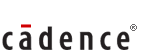Posted by :
Rajesh.So
(19)
Paper: Cadence Placement Paper 2006 (Technical)

1. A sequence is given. You should find out error & write correct 1 2 5 10 13 26 29 48.

ans: Error is 48,there you should write 58.

2. 2,3 6,7 14,15 29,46. Error is 46. In every pair you add 1 to get second number. Ans:29+1=30

3. A girl is 13\'th highest,13th lowest. How many member.

Ans:25

4 .Rearrange MERGANY

Ans: GERMANY  (country)

5. Rearrange BBIRAT

Ans:RABBIT

6. there are 6 persons k,l,m,n,o,p to give seminar cond: 3 persons should give before lunch and 3 after lunch l should immediately precede the seminar of m there should be no gap between l and m .....

ans: 1. L position is 2
2 m pos is 5
3. k pos is 4

7. A is daughter of Y, Z is father of Y. Q is son of Z,
1.if T is son of Y, then
ans: T and A brother/sister
2. if m is brother of T, then
ans :Z is grandfather of M and M & A are brother/sister
3. if Q is son of Z them
ans: Q & Y are brothers
Q. if speed of ongoing train is 24 and while return is 48
avg = ----

ans:32

8. 0.23 + 0.02323 + 0.002323.... == ???

9. 0.4 + 0.44 + 0.444 ..... = ???

10. 1,2,2,4,8,?

ans:32

11. 55*55*55+45*45*45/55*55-55*45+45*45

ans:100

12. 4^^61 + 4^^62 + 4^^63 + 4^^64 . this sum is divisible by which of the following ?

ans:17